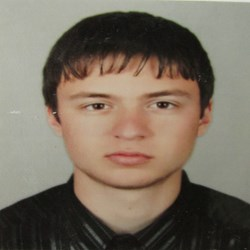tiapko 31 Точки

## Methods and Debugging -> Debugging and Program Flow -> 8. Multiply Evens by Odds

Здравейте,

Можете ли да ми кажете къде бъркам в тази задача или по скоро в решението и, че нещо не мога да разбера.

## 1.Multiply Evens by Odds

Create a program that reads an integer number and multiplies the sum of all its even digits by the sum of all its odd digits:

### Examples

 Input Output Comments 12345 54 12345 has 2 even digits - 2 and 4. Even digits has sum of 6. Also it has 3 odd digits - 1, 3 and 5. Odd digits has sum of 9. Multiply 6 by 9 and you get 54. -12345 54

http://pastebin.com/aQfNYw3N

Тагове:
0NetSpeed 3 Точки

Ето това е кратко решение. Няма нужда да се дефинират много методи, при положение че операцията четни/нечетни може да се изгради чрез един if().

https://pastebin.com/rD22sEEU

1